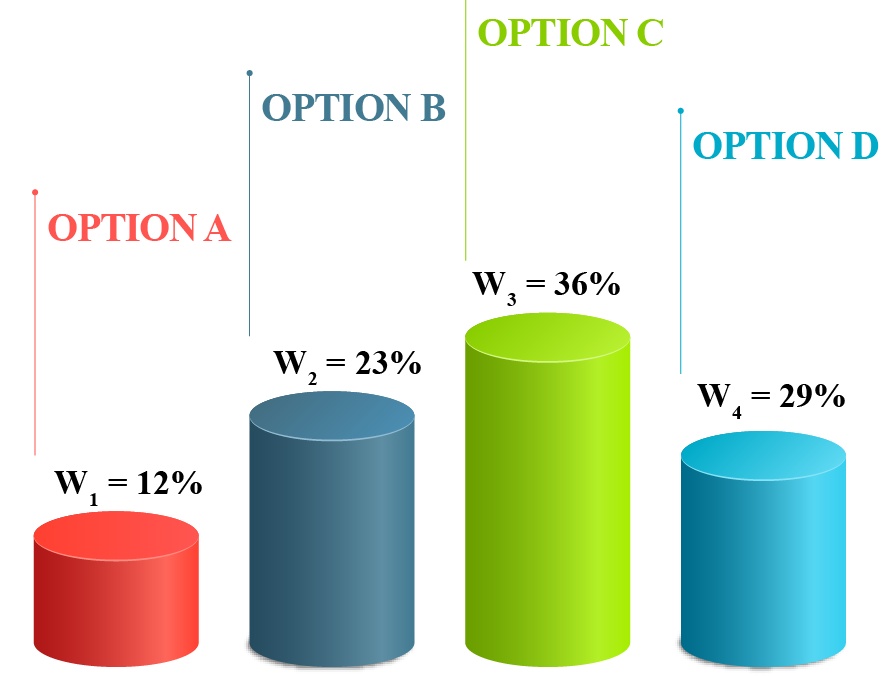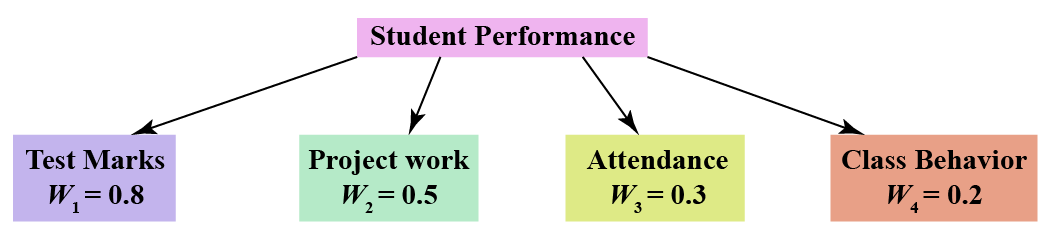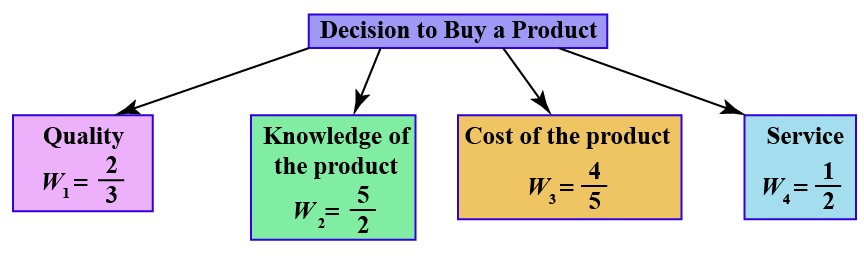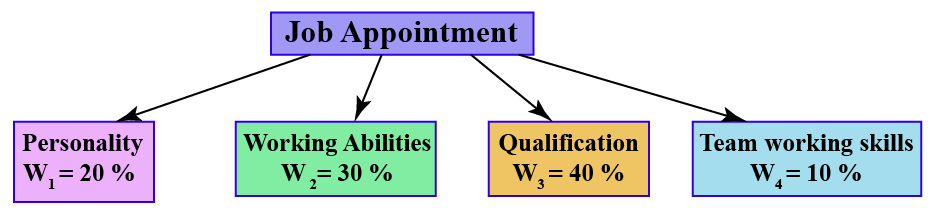# weighted average

weighted average

The weighted average is helpful to make a decision when there are many factors to consider and evaluate.

Each of the factors is assigned some weights based on their level of importance, and then the weighted average is calculated using a mathematical formula.

The weights do not have any physical units and are only numbers expressed in percentages, decimals, or integers.In this mini-lesson, we shall explore the topic of weighted average, by finding answers to questions like what is the meaning of weighted average, what are the examples of weighted average, and how to find the weighted average.

## Lesson Plan

 1 What Is the Meaning of Weighted Average? 2 Important Notes on Weighted Average 3 Thinking Out of the Box! 4 Solved Examples on Weighted Average 5 Interactive Questions on Weighted Average

## What Is the Meaning of Weighted Average?

The weighted average assigns certain weights to each of the individual quantities.

The weighted average formula is the summation of the product of weights and quantities, divided by the summation of weights.

$$\text{Weighted Average} = \dfrac{\sum (\text {Weights} \times \text {Quantities})}{\sum \text {Weights}}$$

Assigning weights would mean different levels of importance to different quantities.

## What Are the Examples of Weighted Average?

A few of the daily life examples would help us to better understand this concept of weighted average.

• A teacher evaluates a student based on the test marks, project work, attendance, and class behavior. Further the teacher assigns weights to each of the criterion, to makes a final assessment of the performance of the student.• A customer's decision to buy or not to buy a product depends on the quality of the product, knowledge of the product, cost of the product, and service by the franchise. Further, the customer assigns weight to each of these criteria and calculates the weighted average.  This will help him in making the best decision.• For appointing a person for a job, the interviewer looks at his personality, working capabilities, educational qualification, and team working skills. Based on the job profile, these criteria are given different levels of importance(weights) and then the final selection is done.## How to Calculate Weighted Average?

The weighted average formula is the summation of the product of the weights and quantities, divided by the summation of the weights.

$\bar x = \frac{w_1x_1 + w_2x_2 + ......+ w_nx_n}{w_1 + w_2 + ... + w_n}$

$\bar x = \frac{\sum w_nx_n}{\sum w_n}$

### Example

Let us understand this calculation of weighted average with the help of an example.

The below table presents the weights of different decision features of an automobile.

With the help of this information, we need to calculate the weighted average.

Quantity Weight
Safety - 8/10  40%
Comfort - 6/10  20%
Fuel mileage - 5/10 30%
Exterior looks - 8/10 10%

Let us now calculate the final rating of the automobile using the concept of weighted average.

\begin{align} \text{Weighted Average} &= 40\%\times \frac{8}{10} + 20\% \times \frac{6}{10} + 30\% \times \frac{5}{10} + 10\% \times \frac{8}{10} \\ &= 0.4\times 0.8 + 0.2 \times 0.6 + 0.3 \times 0.5 + 0.1 \times 0.8 \\ &= 0.32+0.12 + 0.15 + 0.08 \\ &= 0.67 = 6.7/10 \end{align}

The overall rating of the car is 6.7/10

More Important Topics
Numbers
Algebra
Geometry
Measurement
Money
Data
Trigonometry
Calculus
More Important Topics
Numbers
Algebra
Geometry
Measurement
Money
Data
Trigonometry
Calculus
Learn from the best math teachers and top your exams

• Live one on one classroom and doubt clearing
• Practice worksheets in and after class for conceptual clarity
• Personalized curriculum to keep up with school